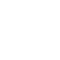# Checksum Calculation at the Sender / Receiver

The error detection method used by most TCP/IP protocols is called the checksum. The checksum protects against the corruption that may occur during the transmission of a packet. It is redundant information added to the packet.

The checksum is calculated at the sender and the value obtained is sent with the packet. The receiver repeats the same calculation on the whole packet including the checksum. If the result is satisfactory (see below), the packet is accepted; otherwise, it is rejected.

#### Checksum Calculation at the Sender

At the sender, the packet header is divided into n-bit sections (n is usually 16). These sections are added together using one’s complement arithmetic (see Appendix D), resulting in a sum that is also n bits long. The sum is then complemented (all 0s changed to 1s and all 1s to 0s) to produce the checksum.

To create the checksum the sender does the following:

• The packet is divided into k sections, each of n bits.
• All sections are added together using one’s complement arithmetic.
• The final result is complemented to make the checksum.

#### Checksum Calculation at the Receiver

The receiver divides the received packet into k sections and adds all sections. It then complements the result. If the final result is 0, the packet is accepted; otherwise, it is rejected. Figure shows graphically what happens at the sender and the receiver.

We said when the receiver adds all of the sections and complements the result, it should get zero if there is no error in the data during transmission or processing. This is true because of the rules in one’s complement arithmetic.

Assume that we get a number called T when we add all the sections in the sender. When we complement the number in one’s complement arithmetic, we get the negative of the number. This means that if the sum of all sections is T, the checksum is T.When the receiver receives the packet, it adds all the sections. It adds T and T which, in one’s complement, is 0 (minus zero). When the result is complemented, 0 becomes 0. Thus if the final result is 0, the packet is accepted; otherwise, it is rejected.#### Checksum in the IP Packet

The implementation of the checksum in the IP packet follows the same principles discussed above. First, the value of the checksum field is set to 0. Then, the entire header is divided into 16-bit sections and added together. The result (sum) is complemented and inserted into the checksum field.

The checksum in the IP packet covers only the header, not the data. There are two good reasons for this. First, all higher-level protocols that encapsulate data in the IP datagram have a checksum field that covers the whole packet.

Therefore, the checksum for the IP datagram does not have to check the encapsulated data. Second, the header of the IP packet changes with each visited router, but the data do not. So the checksum includes only the part that has changed. If the data were included, each router would have to recalculate the checksum for the whole packet, which means an increase in processing time.

Checksum in IP covers only the header, not the data.

Example : Figure shows an example of a checksum calculation at the sender site for an IP header without options. The header is divided into 16-bit sections. All the sections are added and the sum is complemented. The result is inserted in the checksum field.Example : Figure shows the checking of checksum calculation at the receiver site (or intermediate router) assuming that no errors occurred in the header. The header is divided into 16-bit sections. All the sections are added and the sum is complemented. Since the result is 16 0s, the packet is accepted.